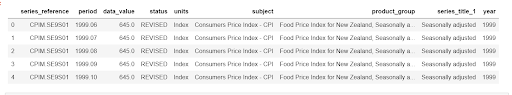How to find top 10 rows by random sampling using pandas?
BIG DATA RECIPES DATA CLEANING PYTHON DATA MUNGING MACHINE LEARNING RECIPES PANDAS CHEATSHEET     ALL TAGS

# How to find top 10 rows by random sampling using pandas?

This recipe helps you find top 10 rows by random sampling using pandas

0

## Recipe Objective

In most of the big data scenarios , random sampling is a method used to select the objects in the data in which there is an equal probability of selecting any particular item. Here, each number has an equal probability of getting picked. Random sampling method is used to give an unbiased representation of the population. In this recipe we are sampling data based on certain ranking criteria and picking top 10 rows after sampling the data.

## System requirements :

• Install the python module as follows if the below modules are not found:
• `pip install pandas`
• `pip install numpy`
• The below codes can be run in Jupyter notebook, or any python console

## Step 1: Import the module

In this scenario we are going to use pandas numpy and random libraries import the libraries as below :

``` import pandas as pd import random import numpy ```

## Step 2 :Prepare the dataset

Here we are using the food related comma separated values dataset to perform the csv file.

Data of Output looks as follows:Reading the csv file from my local using pandas library as below and storing data as dataframe.

``` path ='C:\\Users\\DELL E7440\\Desktop\\food_prices.csv' df = pd.read_csv(path) ```

After loading the csv file into dataframe filter the dataframe for submitted "year" records and load into the dataframe as below

``` df = df[(df["status"] == 'REVISED')] ```

Creating a random number columns for a ranking criteria follow as below

``` df['random_between'] = df.apply(lambda row: random.randint(1, row.year), axis=1) ```

A new random number is generated which is an addition of the above 2 columns as a separate column.

``` df['random_number'] = df['random_between'] + df['random'] ```

The above random number is used as a ranking criterion for the data which is grouped by the 'series_reference' column.

``` df_grouped = df.groupby(['series_reference']) ```

Rank the rows in each group, based on the generated random numbers

``` df['Rank'] = df_grouped['random'].rank(method='min') ```

The rows which have a rank < 11 are captured into the final results data frame.

``` df = df[(df.Rank<11)] ```

Completed code as written together in function to get the random records as follows:

``` import pandas as pd import random import numpy def get_food_data_submission(): # Initialize the client to run the query path='C:\\Users\\DELL E7440\\Desktop\\food_prices.csv' try: # read and Convert the output to Pandas Dataframe df = pd.read_csv(path) # Filter the dataframe for Submitted Records df = df[(df["status"] == 'REVISED')] # Create random number columns for a ranking critiria df['random_between'] = df.apply(lambda row: random.randint(1, row.year), axis=1) df['random'] = df.apply(lambda row: random.uniform(0, 1), axis=1) df['random_number'] = df['random_between'] + df['random'] # Group the data by 'series_reference' df_grouped = df.groupby(['series_reference']) # Rank the rows in each group, based on the generated random numbers df['Rank'] = df_grouped['random'].rank(method='min') # Fetch the top 10 records submitted by the year df = df[(df.Rank<11)] return df except Exception as exp: print("Error occured, ", exp) # calling the function to return the results get_food_data_submission() ```

Output of the above code as follows:#### Relevant Projects

##### Hive Project - Visualising Website Clickstream Data with Apache Hadoop
Analyze clickstream data of a website using Hadoop Hive to increase sales by optimizing every aspect of the customer experience on the website from the first mouse click to the last.

##### Real-Time Log Processing using Spark Streaming Architecture
In this Spark project, we are going to bring processing to the speed layer of the lambda architecture which opens up capabilities to monitor application real time performance, measure real time comfort with applications and real time alert in case of security

##### Event Data Analysis using AWS ELK Stack
This Elasticsearch example deploys the AWS ELK stack to analyse streaming event data. Tools used include Nifi, PySpark, Elasticsearch, Logstash and Kibana for visualisation.

##### Real-Time Log Processing in Kafka for Streaming Architecture
The goal of this apache kafka project is to process log entries from applications in real-time using Kafka for the streaming architecture in a microservice sense.

##### Web Server Log Processing using Hadoop
In this hadoop project, you will be using a sample application log file from an application server to a demonstrated scaled-down server log processing pipeline.